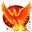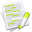#wx.lib.floatcanvas.FCObjects.Arrow¶

Draws an arrow

It will draw an arrow , starting at the point XY points at an angle defined by Direction.

##Class Hierarchy¶Inheritance diagram for class Arrow:

##Methods Summary¶

 __init__ Default class constructor. CalcArrowPoints Calculate the arrow points. SetDirection Set the direction SetLength Set the length SetLengthDirection Set the length and direction

##Class API¶

class Arrow(XYObjectMixin, LineOnlyMixin, DrawObject)

Draws an arrow

It will draw an arrow , starting at the point XY points at an angle defined by Direction.

### Methods¶

__init__(self, XY, Length, Direction, LineColor = "Black", LineStyle = "Solid", LineWidth = 2, ArrowHeadSize = 8, ArrowHeadAngle = 30, InForeground = False)

Default class constructor.

Parameters
• XY – the (x, y) coordinate of the starting point, or a 2-tuple, or a (2,) NumPy array

• Length (integer) – length of arrow in pixels

• Direction (integer) – angle of arrow in degrees, zero is straight up + angle is to the right

• LineColor – see SetColor

• LineStyle – see SetLineStyle

• LineWidth – see SetLineWidth

• InForeground (boolean) – should object be in foreground

CalcArrowPoints(self)

Calculate the arrow points.

SetDirection(self, Direction)

Set the direction

Parameters

Direction (integer) – angle of arrow in degrees, zero is straight up + angle is to the right

SetLength(self, Length)

Set the length

Parameters

Length (integer) – length of arrow in pixels

SetLengthDirection(self, Length, Direction)

Set the length and direction

Parameters
• Length (integer) – length of arrow in pixels

• Direction (integer) – angle of arrow in degrees, zero is straight up + angle is to the right